﻿
Reach Us+44-1522-440391
Enhancement of the Resolution of CCD Undersampling with Amplitude Grating and Subpixeling

# Journal of Lasers, Optics & PhotonicsOpen Access

All submissions of the EM system will be redirected to Online Manuscript Submission System. Authors are requested to submit articles directly to Online Manuscript Submission System of respective journal.

# Enhancement of the Resolution of CCD Undersampling with Amplitude Grating and Subpixeling

Sohail M*
Department of Physics, University of Lahore, Punjub, Pakistan
*Corresponding Author: Sohail M, Department of Physics, University of Lahore, Panjub, Pakistan, Tel: +92 (0)42 111-865-865, Email: [email protected]

Received Date: Apr 09, 2018 / Accepted Date: May 23, 2018 / Published Date: May 30, 2018

Keywords: Super-resolution; Charge coupled device; Spatial resolution; Amplitude grating; Subpixeling

#### Introduction

An optical imaging system is composed of two main parts: the optical part and the electronic part. Simplest optical system may contain a single well corrected diffraction limited lens as the key element . A lens with a diameter D and focal length f imaging a scene at a distance u from the lens using wavelength λ can resolve the two point objects at the imaging plane provided the separation between them at the object plane exceeds 1.22λ..u / D. This provides the classical Rayleigh Resolution limit to an optical imaging system consisting of a single lens . The electronic part of the imaging system is composed mainly of CCD (charged coupled device) camera chip which is placed at the imaging plane of the optical system to acquire the image electronically . The CCD chip has two parameters that play a role in the resolution of the imaging system. One is the nonzero size of the pixels and other is the separation between the pixels [4,5]. The object information contained in the area of dimensions Δxo by Δyo (referred to as object pixel) is mapped to a CCD pixel of size Δx by Δy through the relation: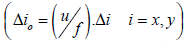where “u” is the distance between the sensor and the object. A CCD pixel integrates all the information falling on the pixel area and so the object information contained in the object pixel is lost. This type of loss of resolution due to a finite non-zero CCD pixel size can only be avoided provided the CCD pixels follow the condition Δ ≤ 0.61.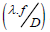. This condition can be met only in certain situation where the lens aperture is small and the focal length is large. Such situations are not practicable and so the condition is hard to realize. This means that the resolution is mainly limited by the finite CCD pixel size and this type of resolution limit is known as Geometric Resolution Limits (GRL) [6,7]. Techniques to remove this GRL are known as Geometric Super-Resolution (GSR) techniques. Subpixeling techniques provide a solution to GRL and GSR can be achieved. Optical Mask technique can also be used to achieve GSR.

The second factor of the CCD that limits the GRL is the separation between the pixels . This can somewhat be overcome by using fill factor approaching 100% in which the separation between the pixels is negligibly small in comparison with pixel dimensions. There are situations where 100% fill factor is not possible to achieve as in case of sparse array detectors and the separation between the pixels should be such that they satisfy the Nyquist Sampling Theorem . In situations where the sampling theorem cannot be satisfied, there are still some possibilities to obtain GSR by using optical masks at the Fourier plane in a 4f optical system. The under-sampled image captured by the CCD is Fourier transformed and the same optical mask is used to nullify the effect of under-sampling by the CCD. The researchers have dealt the two geometric resolution limiting factors separately. Previous cases of under-sampling by the CCD have been dealt in literature assuming point pixels in which the width of the CCD pixels is assumed to be nearly zero [9-12].

To our knowledge, we for the first time are combining the resolution limits due to non-zero pixels size and the under-sampling by the CCD pixels simultaneously for the improvement of geometric super-resolution. For simplicity, a 4f imaging system will be used to establish the concept. Calculation and simulations will be presented in one dimension. An optical mask will be placed at the Fourier plane in a 4f system and an image will be captured by an under-sampled CCD placed at the imaging plane. A mask attached to the CCD will be moved in sub-pixel steps and some under-sampled images will be recorded.

The CCD under-sampled images will be combined according to the method reported by Haq I ul and Mudassar . The resulting image is Fourier transformed and the same optical mask will be used at the Fourier spectrum to remove the overlapping between the copies of the original spectrum which is inverse Fourier transformed to obtain a high resolution image. These steps will improve the GSR and optical SR will not be discussed.

In section 2 mathematical modeling of the proposed technique will be presented. In section 3 simulation results will be presented. Section 4 will conclude the paper.

#### Mathematical Modeling

Let the input object be represented by the function g(x) placed at the input plane in a 4f system. Let G(v) represents the Fourier transform of the g(x), we can simply write the following relation.

G(v) = ℑ{g(x)} (1)

The “Optical mask” (referred to as the encoding mask) OM(v) is multiplied to the object spectrum G(v), and the resulting spectrum O(v) becomes,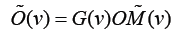(2)

The simplest optical mask may consist of a series of delta function. We are using the coordinate “v” to represent the Fourier plane and the coordinate “x” to represent both the object and image plane coordinates. The mathematical representation of the optical mask can be given by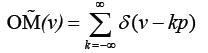(3)

Where p is the spacing between the two consecutive delta peaks or the period of the encoding mask and “k” is the running integer. The spectrum after multiplication by the encoding mask can be written as: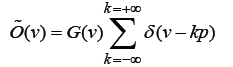(4)

Considering a band-limited input with spectrum width equal to 2ΔV the number of points sampled by the mask will be N=2ΔV/p.

The second lens in the 4f system takes the inverse Fourier of the spectrum given by eqn. (4) and the image at the image plane of the 4f system can be written as: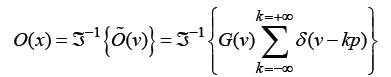(5)

A CCD is placed at the image plane which captures the image. The image is under-sampled by the CCD pixels. Here we are considering each pixel of width equal to 2Δx. A CCD function in one dimension (CCD(x)) can be formed by convolving each point pixel with a rectangular function of width 2Δx and is given by: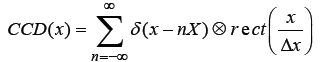(6)

X” is the spacing between the center of the CCD pixels or the pixel period and to ensure under-sampling by the CCD, the required conditions is: X ≥ 1 / (2ΔV+ p). The image O(x) is sampled by the CCD(x) and the sampled image S(x) can be written by the following equation.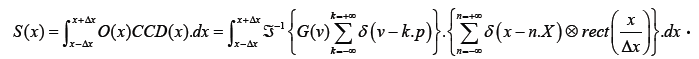(7)

For the Discrete case, the integral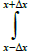can be replaced by another summation sign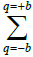if each pixel of width 2Δx is divided into (2b+1) sub-pixel each of width 2.δx, rect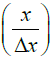can be replaced by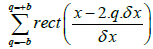, then the above equation takes the following form.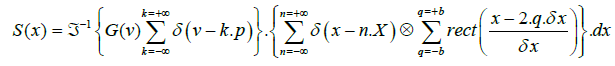(8)

In eqn. (7) the image information falling on each pixel of width 2Δx is averaged out and the image detail on each pixel is lost. To recover the image detail information falling on each pixel, sub-pixeling is performed with a width of each sub-pixel equal to 2.δx. Here we are assuming that the sub-pixels perform Nyquist Sampling of the image information falling on them. The highest frequency in the image (fmax) is then restricted by the following relation,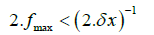(9)

O(x) contains multiple replicas of the original object due to the sampling at the Fourier plane by the optical mask and S(x) is the undersampled versions of O(x). Due to the integration of data at each pixel of the CCD (eqn. (7)) the object information is lost which is not recoverable by any means except that a sub-pixeling technique is required in which a pixel of size 2 Δx is divided into (2b+1) sub pixels each of size 2.δx using a three level mask (0.50,100) at the CCD plane as explained in Harrias . The subpixeling should be such that the information falling on each pixel can be recoverable as restricted by eqn. (9). Under such a condition the integral in eqn. (7) can be removed and the eqn. (7) will take the form as given by eqn. (8). Due to the under-sampling by the CCD, the Fourier transform of eqn. (8), contains multiple copies of the spectrum of the original object. The spectral copies overlap due to under-sampling by the CCD as shown in the Figure 1. The Fourier transform of eqn. (8) is given below.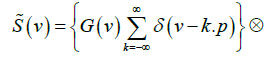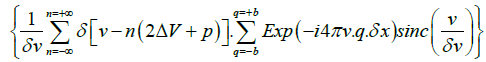(10)

Where ‘ ⊗ ’ denotes convolution operation. In the simplification of eqn. (10), we have used the following relationships: 1/X=2ΔV+p. For simplicity of calculations we assume that an integer number of subpixels fit into the separation between the two consecutive pixels, i.e., X = m.δ x or 1 / X = 1 / mδ x which may be written mathematically as: δ v = m.ΔV or δ v >> ΔV where δv =1/δx and Equation (10) becomes: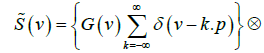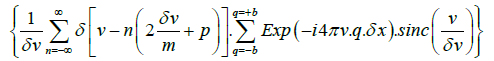(11)

Using the convolution property: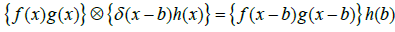Eqn. (11) takes the following form.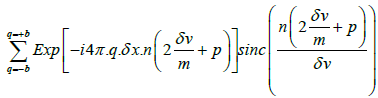(12)

To remove the overlapped copies of spectrum in eqn. (12), it is multiplied with the decoding mask (same as the encoding mask) and the resultant equation becomes: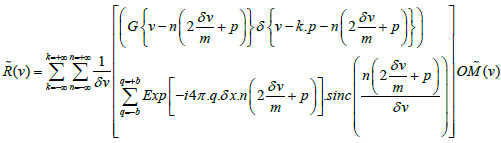(13)

Using the optical mask from eqns. (3), and (13) becomes: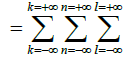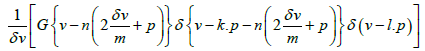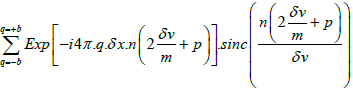(14)

The decoding mask is placed exactly where the encoding mask was placed in which case we can set l.p=k.p+n [(2δv/m)+p] for even values of n and the two delta functions in eqn. (14) are simplified as given: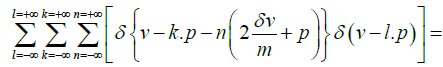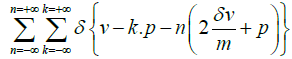(15)

Using eqn. (15) into eqn. (14), the resultant equation takes the following form.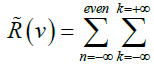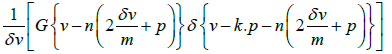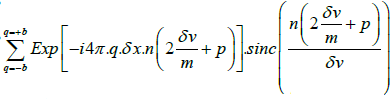(16)

Eqn. (16) provides the un-overlapped copies of the spectrum as shown in the Figure 2:

Figure 2: Un-overlapped spectra of the CCD undersampled data after using the decoding mask.

To select the central copy we can set n=0 and eqn. (16) takes the form given below.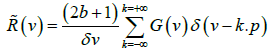(17)

Eqn. (17) gives the original copy of the object’s spectrum when normalized but sampled at points separated by the mask period. The missing points or the holes in the spectrum are obtained through the interpolation. The synthesized spectrum is then inverse Fourier transformed to obtain the image free of aliasing. The mathematics of the proposed technique has established that by using encoding and identical decoding masks at the Fourier plane and subpixeling at the CCD plane we can obtain aliased free data with the use of under-sampling device like CCD consisting of pixels with non-zero dimensions.

#### Simulation Results and Discussions

For the simulation of proposed technique we have chosen a simple Gaussian shaped object as the input object for simulation which is shown in Figure 3a. The vertical axis represents the intensities of the object at different points and the horizontal axis for the spatial data points of the object in one dimension. The Fourier spectrum of the input in Figure 3a is shown in Figure 3b. The vertical axis represents the intensity of the image while the horizontal axis gives the data points of the image. The spectrum shown in Figure 3b will appear at the back focal plane (Fourier transform plane) of the Fourier transforming lens in a 4f system. An optical mask consisting of delta functions occurring at alternate positions (period p=2, eqn. (3)) is shown in Figure 3c (a small magnified portion is shown only) is placed at the Fourier transform plane in a 4f system. The optical mask samples the spectrum shown in Figure 3b and the sampled band limited spectrum is shown in Figure 3d. The second lens of the 4f system takes the inverse Fourier of the sampled spectrum and the image at the imaging plane of the 4f system will appear as shown in Figure 3e. The image in Figure 3e consists of two copies of the original object being the inverse Fourier of the sampled original spectrum at alternate points. A CCD camera is placed at the imaging plane of the 4f system with finite pixel size equal to 2 au (arbitrary units). The data falling on each pixel is integrated and the data bits at a pixel are lost and only integrated data is recovered. To recover the lost data bits falling on each CCD pixels, a subpixeling is required. The subpixeling follows the method . Due to the application of technique  the subpixel data is recovered and is shown in Figure 3g. Here we have assumed that the pixels are located far from each other (by 18 pixels apart) so that the data sampled by the CCD is undersampled. The CCD pixels of width 2 (au) separated by 18 (au) is shown in Figure 3f. The under-sampled image after application of subpixeling algorithm is shown in Figure 3g. The Fourier spectrum of the image in Figure 3g is shown in Figure 3h. This contains 19 full spectral copies of the original spectrum with the non-zero spectrum width equal to 54 pixels. The spectral copies overlap as obvious from the Figure 3h. The spectral copies shown in Figure 3h are now multiplied with the decoding mask (same as the encoding mask). The decoding mask removes the spectral overlap and the un-overlapped spectral copies are shown in Figure 3i. The spectral copies are multiplied by the weighting factor according to eqn. (16) except the central copy which is obtained by setting n= 0 in eqn. (16). The filtered spectrum copy is shown in Figure 3j. This is the same as the original copy of spectrum shown in Figure 3d except that data is on different scale. The recovered spectrum shown in Figure 3j has holes at alternate points. The data at the missing points is obtained through standard interpolation techniques and the spectrum after filling the holes is shown in Figure 3k. The inverse Fourier of the data shown in Figure 3k gives the original object which is shown in Figure 3l after normalization. The recovered object shown in Figure 3l is the same as the original object shown in Figure 3a to within computational errors. The image taken by the CCD without using subpixeling and Optical Mask is shown in Figure 3m shows that most of the object information was lost. Thus our presented technique has recover most of the information of the object which was lost due the CCD pixels separation as well as pixel dimension.

Figure 3: (a): Input Object, (b): Fourier transform of the input object, (c) :Optical Mask, (d): Multiplication of (b) and (c), (e): Inverse of (d) is the Image which is at the CCD plane, (f): CCD, (g): undersample image of the CCD after subpixeling , (h): Fourier transform of (g), (i): Multiplication of Optical mask and (h), (j): Multiplication of the Filter with (i) to select the central copy of the spectrum, (k): Interpolation of (j), (l): Inverse of (k) gives the Output Image, (m): image obtain without using subpixeling and optical mask.

The proposed technique also presented in two dimension using Lena image which is more complex than Gaussian function as input object shown in Figure 4a. The Fourier spectrum of input appeared at back focal plane of the objective lens where the optical mask placed. The optical mask sampled this spectrum and inverse Fourier transformation of this coded spectrum gives image of the input at the CCD plane shown in Figure 4b. CCD sampled the image shown in Figure 4b and 4c shows the spectrum of the sampled image. Figure 4c was multiplied with the Optical Mask that vanishes the overlapping effect shown in Figure 4d. A single Spectrum is selected from the unoverlapped spectra using suitable rectangular filter and interpolation technique is used to fill the missing data of the spectrum shown in Figure 4e and 4f is the inverse Fourier transforms of the Figure 4e. Which is approximately similar to the input object? The image shown in Figure 4g is the output image obtains by using conventional imaging system. It is clear that our approach has enhanced the resolving power of the imaging system.

Figure 4: (a): Input Object, (b): Image at the CCD plane, (c): Fourier transform of image sampled by CCD (d): Multiplication of Optical mask and (c), (e): Multiplication of the Low pass Filter with (d) to select the central copy of the spectrum and Interpolation, (f): Inverse of (e) gives the Output Image, (g): Output image obtain without using subpixeling and optical mask.

#### Conclusion

Most previous geometric super resolution techniques which have used optical filters at the Fourier plane, mechanical elements for the motion of the CCD etc. But here we have used the optical mask at the Fourier plane that encodes the object spectrum with a rectangular aperture. The objective lens produces the image of the coded spectrum at the CCD plane and the CCD capture the image. In this work we have assumed rectangular shaped pixels of the CCD having some dimension. Other techniques dealt with sub-pixeling of CCD pixels and have assumed non-zero sized pixels. In this paper we have gathered the two techniques in which we deal with both the under sampling by an imaging device like CCD and at the same time consider the nonzero sized pixels. In subpixeling the mask is moved in a subpixel step and the intensities was recorded while the scene and the CCD remain stationary. Due the subpixeling we have resolve the information very smaller than the pixel size and so the resolution was enhanced. The under sampling by CCD was solved by placing an optical mask at the Fourier plane in a 4f system. This work has overcome two problems, pixel pitch and pixel dimension i.e., the integration of data by nonzero sized pixels. Mathematical calculation and the simulation results have presented to support our idea and have tried to establish that the proposed technique seems to work as far as the simulation is concerned.

#### Acknowledgements

We are thankful to Higher Education Commission of Pakistan (HEC) for funding all our projects for providing research facilities.

#### References

Citation: Sohail M (2018) Enhancement of the Resolution of CCD Undersampling with Amplitude Grating and Subpixeling. J Laser Opt Photonics 5: 185. DOI: 10.4172/2469-410X.1000185

Copyright: © 2018 Sohail M. This is an open-access article distributed under the terms of the Creative Commons Attribution License, which permits unrestricted use, distribution, and reproduction in any medium, provided the original author and source are credited.

Select your language of interest to view the total content in your interested language

##### Recommended Journals
Viewmore
###### Article Usage
• Total views: 3247
• [From(publication date): 0-2018 - Sep 22, 2019]
• Breakdown by view type
• HTML page views: 3185

## Post your commentCan't read the image? click here to refresh
###### Peer Reviewed Journals

Make the best use of Scientific Research and information from our 700 + peer reviewed, Open Access Journals

International Conferences 2019-20

Meet Inspiring Speakers and Experts at our 3000+ Global Annual Meetings

Top